### 【数据结构】什么是AVL树

• 2019 年 10 月 3 日
• 筆記

# 什么是AVL树

## 1. 什么是AVL树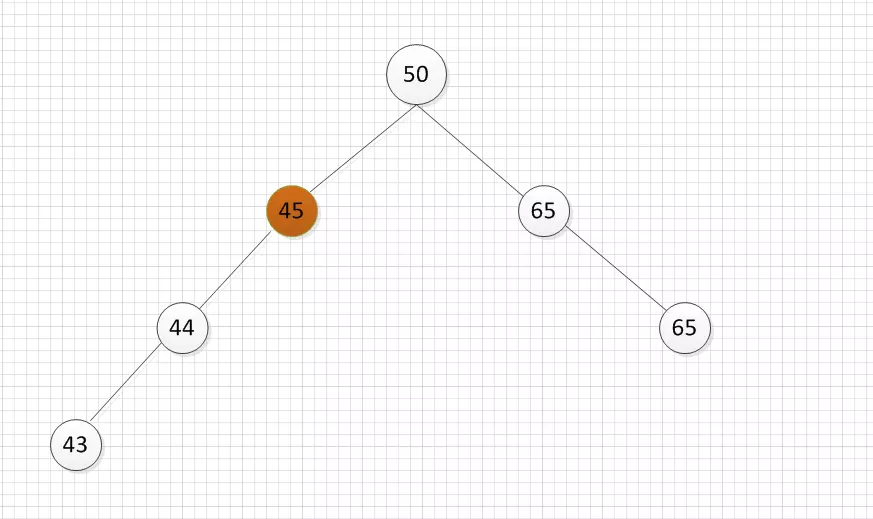## 2. 节点的实现

``public class AVLTreeNode<T extends Comparable> {        // 存储的数据-用于排序      T key;        // 节点高度-用于计算父节点的BF      int height;        // 左儿子 & 右儿子      AVLTreeNode<T> left;      AVLTreeNode<T> right;        public AVLTreeNode() {      }        public AVLTreeNode(T key, AVLTreeNode<T> left, AVLTreeNode<T> right) {          this.key = key;          this.left = left;          this.right = right;          this.height = 0;      }    }  ``

``public class AVLTree<T extends Comparable> {      // 定义树的根节点      AVLTreeNode<T> root;        public AVLTree() {          root = null;      }  }  ``

``    public int height() {          return height(root);      }        private int height(AVLTreeNode<T> tree) {          if (tree != null){              return tree.height;          }          return 0;      }  ``

## 3. AVL树的调整

• 对a的左儿子的左子树进行一次插入。（LL）
• 对a的左儿子的右子树进行一次插入。（LR）
• 对a的右儿子的左子树进行一次插入。（RL）
• 对a的右儿子的右子树进行一次插入。（RR）
其中1、4是关于a点的镜像对称，2、3是关于a点的镜像对称。

### 3.1 LL旋转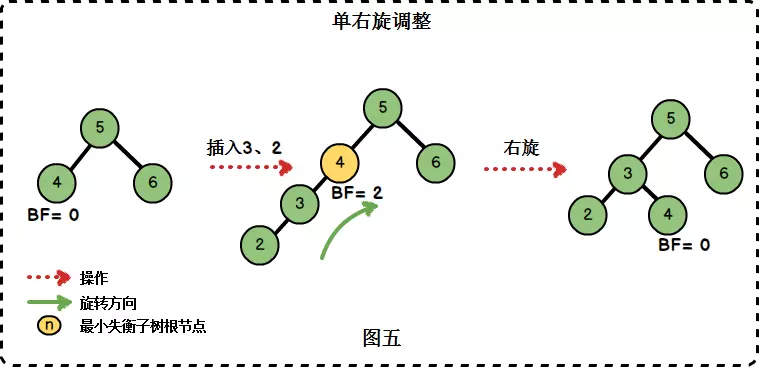``    /**       * 进行一次单右旋转       *       * @param node 最小失衡树根节点       */      private AVLTreeNode<T> rightRotation(AVLTreeNode<T> node) {          AVLTreeNode<T> left = node.left;          node.left = left.right;          left.right = node;          // 更新高度          node.height = Math.max(height(node.left), height(node.right)) + 1;          left.height = Math.max(height(left.left), height(left.right)) + 1;          return left;      }  ``

### 3.2 RR旋转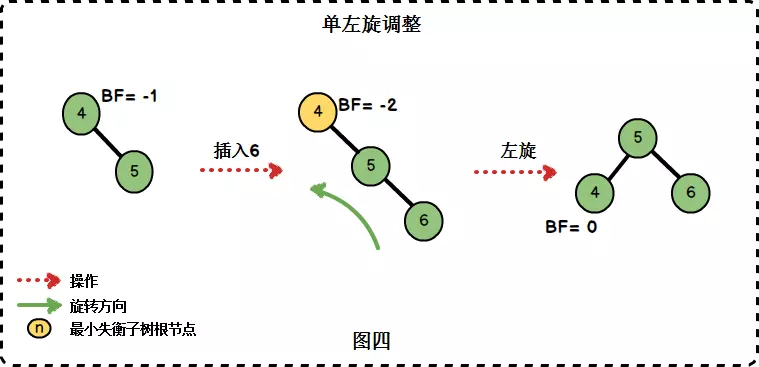``    /**       * 进行一次单左旋转       *       * @param node 最小失衡树根节点       */      private AVLTreeNode<T> leftRotation(AVLTreeNode<T> node) {          AVLTreeNode<T> right = node.right;          node.right = right.left;          right.left = node;            // 更新高度          node.height = Math.max(height(node.left), height(node.right)) + 1;          right.height = Math.max(height(right.left), height(right.right)) + 1;            return right;        }  ``

### 3.3 RL旋转

“在右子树上插入左孩子导致AVL树失衡",此时我们需要进行先右旋后左旋的调整。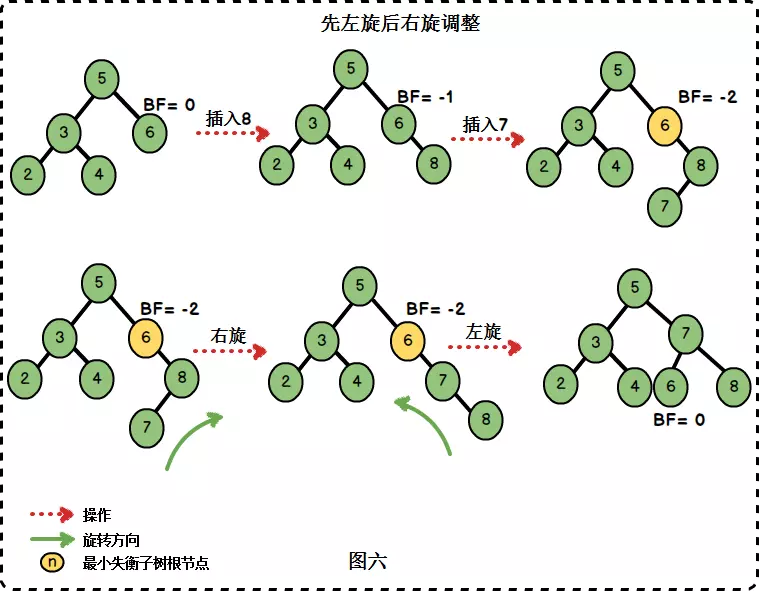``      /**       * 先右旋后左旋       *       * @param node 失衡树根节点       * @return 旋转后的根节点       */      private AVLTreeNode<T> rightLeftRotation(AVLTreeNode<T> node) {          node.right = rightRoation(node.right);            return leftRoation(node);        }    ``

### 3.4 LR旋转

“在左子树上插入右孩子导致AVL树失衡",此时我们需要进行先左旋后右旋的调整。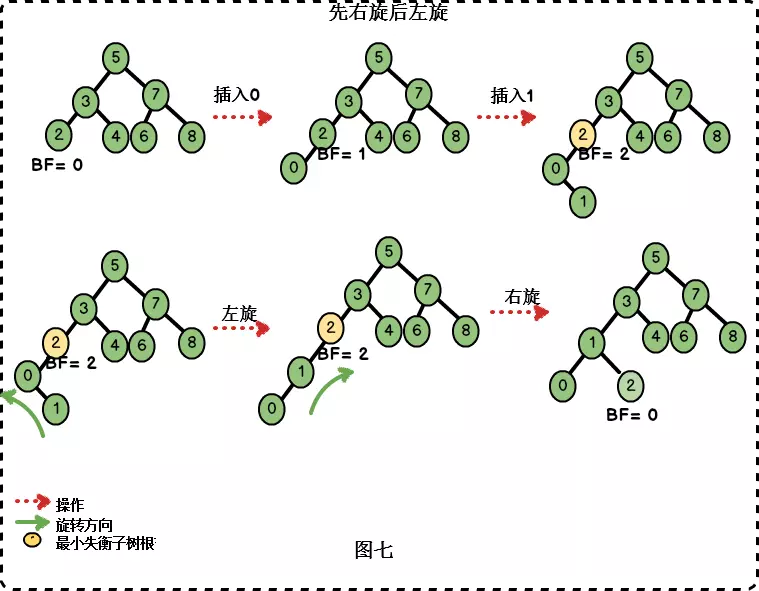``      /**       * 先左旋后右旋       *       * @param node 失衡树根节点       * @return 旋转后的根节点       */      private AVLTreeNode<T> leftRightRotation(AVLTreeNode<T> node) {          node.left = leftRoation(node.left);            return rightLeftRoation(node);        }      ``